ET online
March 2017
Power quality effects on transformers and motors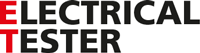Power quality effects on transformers and motors

31 March 2017

Andy Sagl - Product manager

In the first two articles of this series, which can be found by clicking here, we looked at types of power quality problems and how these are categorised and measured. This article follows on by examining how one major power quality problem – harmonics – affects conductors, transformers and motors.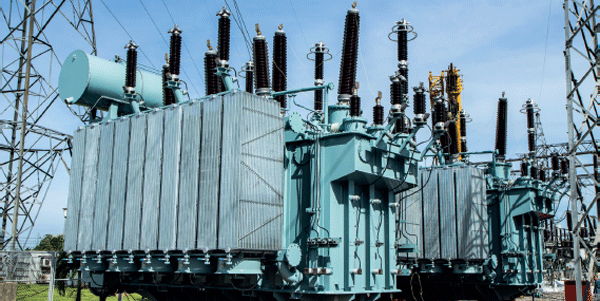Harmonics, heat and neutral conductors

The main problem associated with excessive harmonic distortion can be very simply stated: it gives rise to the generation of heat. Often this is seen as overheating in neutral conductors, transformers and motors.

Let’s look first at neutral conductors. In a system where all phases are balanced and no harmonics are present, the neutral conductor will carry no overall current, because the neutral currents from the three phases cancel each other out. If triplen or zero-sequence harmonics are present, however, the neutral currents associated with these do not cancel each other – instead they add together.

In fact, the more orders of triplen harmonics that are present, all other things being equal, the more current flows in the neutral conductor. For example, the presence of the third harmonic in a given system will produce a certain level of neutral current, but add in, ninth order triplen harmonics and the neutral current will further increase. Since the heating effect is proportional to the square of the current, this can quickly lead to overheating or even failure of the neutral conductor.

Transformers

Harmonics also cause heating effects in transformers. The heating effects can be divided into two principal types: heating due to winding losses (which are also known as copper losses) and heating due to core losses.

As harmonic levels in the supply network continue to increase, due to the spread of non-linear loads such as switching power supplies, there is an ever-growing risk that transformers that have given problem-free service over a long period will start to exhibit excessive heating leading in the worst cases to costly and disruptive failures.

To begin to understand how harmonics cause heating in transformers, let’s start with the simple fact that higher harmonic orders mean higher frequencies. As frequency increases, so does the inductive reactance of a circuit, which means that its total impedance also increases. And, in transformers, this increase in impedance means an increase in winding losses, leading to increased production of heat.

The higher the order harmonic the greater the magnitude of the copper losses and core losses. This happens mostly because of eddy currents, which are circulating currents induced in the conductors of the transformer windings by alternating magnetic fields. The eddy currents produce magnetic fields of their own, which oppose the fields that created them. The repulsion between these opposing fields effectively increases the resistance of the winding, leading to heating and power losses. The higher the frequency of the harmonics, the higher the energy of the eddy currents and the greater their heating effect.

Skin effect

One of the ways in which eddy currents increase the effective resistance of a conductor is known as ‘skin effect’, which is the tendency of AC current flowing in a conductor to distribute itself so that the current density is greatest near the surface of the conductor. We know, eddy currents induced in a conductor produce magnetic fields that oppose the fields that generated them. Within a conductor, these eddy current fields have the effect of forcing current to flow closer to the outside of the conductor, in effect reducing the usable cross-sectional area of the conductor thusly increasing its resistance. This inevitably leads to higher losses and increased heating.

At low frequencies, such as the supply fundamental frequency, the skin effect is minimal, but as the frequency increases, the “skin depth”, in which most of the current flows, becomes smaller and smaller, further increasing conductor resistance and winding loss.

Proximity effect

The proximity effect is another way in which eddy currents lead to increased losses. It is a phenomenon that occurs when an AC current flows through a conductor that is in close proximity to another conductor – a typical example being adjacent turns in a
transformer winding.

As the AC current flows through the first conductor it generates a magnetic field that induces longitudinal eddy currents in the adjacent conductor. The eddy currents tend to constrain the current flow in the second conductor to the side furthest from the first conductor. This once again increases the effective resistance and, as a consequence, losses are also increased and more heat is generated.

Core losses

Our discussion up to this point has focussed on winding or copper losses in transformers, but the presence of harmonics also gives rise to losses in the magnetic core. There are two underlying mechanisms that contribute to these losses: eddy currents and hysteresis.

Eddy currents are induced into the (conductive) material that makes up the transformer core in much the same way that they are induced into the windings. The circulating eddy currents in the core contribute to losses and generate heat. To reduce these effects to a minimum, all practical transformers use laminated cores. The core is made up of thin sheets of transformer steel interleaved with insulating material.

Eddy currents cannot pass through these insulating layers but the magnetic field on which the transformer depends for its operation is affected very little by the presence of the insulating layers. The overall result is that the eddy currents can only circulate within the individual sheets of transformer steel making up the core and are thus greatly reduced, along with their heating effect.

Hysteresis, the second mechanism that contributes to core losses, is associated with the intrinsic behaviour of materials as they are magnetised and demagnetised – specifically, the way the polarity of the magnetic dipoles within the material changes during this process, and the way that magnetic domains form and reform.

It is worth noting that unlike most of the other loss mechanisms in transformers, hysteresis losses are not related to eddy currents. The total core losses, however, are the sum of the hysteresis losses and the eddy current losses.

Transformer de-rating

Because the presence of harmonics increases the losses and heat generated within a transformer it is clear that, if overheating and failure are to be avoided, transformers need to be de-rated according to the level of harmonics they are required to handle. The parameter used for this de-rating is known as the K factor.

In essence, the K factor indicates the level of non-linear load a transformer can tolerate without overheating. Calculation of this factor takes into account the effect of eddy currents created in the windings of the transformer, but not the core losses. The basis for calculating the K factor is the ANSI C57.110 standard which provides the accepted basis for derating transformers operating with non-linear loads. It is worth noting that although ‘K factor’ is accepted as industry-standard terminology, this term is never explicitly mentioned in the standard!

The K factor takes into account the fact that the heating effect of harmonics is proportional to the square of the current and to the square of the harmonic order. A K factor of 1 – which, in effect, describes a standard transformer – means that there are assumed to be no current harmonics present in the supply system. The higher the K factor, the higher the harmonic content the transformer can handle without overheating.

A K factor of 2, for example, indicates that the transformer can handle twice the stray loss heating effects of a standard transformer. In practice, transformers are available with a variety of K factor ratings – typically 4, 9, 13, 20, 30, 40 or 50. Different types of load generate different harmonic components and the transformer K factor should be matched to the type of load. For example, welding equipment may require a transformer with a K factor of 4, whereas a transformer supplying variable speed drives may need a K factor of 20.

The table shows approximate K factors for a variety of loads but is indicative only. A comprehensive K factor analysis, ideally based on power quality determinations made with an accurate power quality instrument, should always be carried out before selecting a  transformer.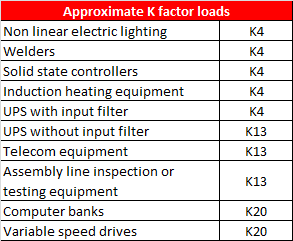Harmonics and motors

The principal effects of harmonics on motors are inefficient operation, overheating and increased levels of vibration. Inefficient operation is the result of negative-sequence harmonics as these produce magnetic fields within the motor that rotate in the opposite direction to the magnetic field resulting from the fundamental supply frequency. This reduces torque and increases the amount of current required by the motor to drive a given load.

The heating effects of harmonics in motors are similar to the heating effects found in transformers. In particular, eddy currents produce the same effects in motor windings and magnetic materials as they do in the windings and magnetic components of transformers. As with transformers, the magnetic components of motors – the stator and rotor formers – are usually laminated to help minimise eddy current losses. Vibration due to harmonics in motors results from the interaction of the magnetic fields produced by the different orders of harmonics. Positive sequence harmonics produce magnetic fields that rotate in the same direction as the magnetic field produced by the fundamental frequency, whereas negative sequence harmonics produce fields that rotate in the opposite direction. The combined effect of these fields can produce vibration in the motor shaft. This is particular problematic when the frequency of the vibration happens to coincide with the mechanical resonant frequency of the shaft. In such cases, the vibrations can build to a large enough amplitude to damage or even destroy the shaft.

Limits and recommendations

Because of the deleterious effects of harmonics on transformers, motors and other assets, standards organisations have laid down limits on the permissible levels of harmonics in the supply system.

The EN50160 limits usually adopted in Europe are shown in Table 1. Note that these limits apply only
to voltage.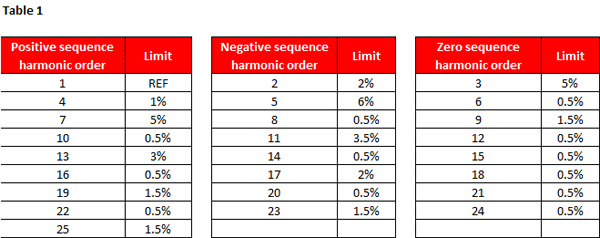No limits are given for harmonics of order higher than 25 as these are usually small, although resonances can make their effects unpredictable.

These are the IEEE519 recommended voltage limits, usually adopted in the USA, as detailed in the 2014 version of the standard. (Table 2)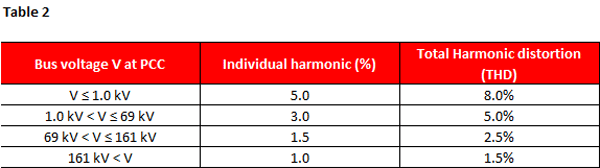Unlike EN50160, the IEEE519 standard includes recommendations for current limits. These are shown in Table 3: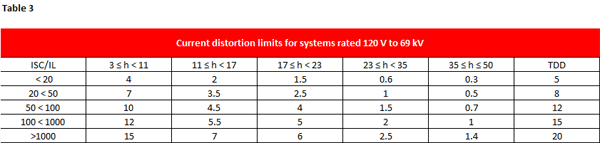Where Isc is the maximum short-circuit current at the point of common coupling (PCC), and IL is the maximum demand load current (fundamental frequency component) at the PCC under normal load operating conditions.

Even harmonics are limited to 25% of the values shown in the table for odd harmonics, and current distortions that result in a DC offset – such as those produced by half-wave converters, for example – are not allowed. All power generation equipment is limited to the values of current distortion shown in the table, irrespective of the actual value of Isc/IL.